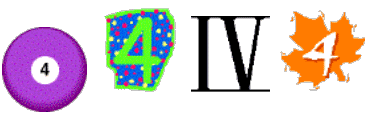#### You may also like### Pebbles

Place four pebbles on the sand in the form of a square. Keep adding as few pebbles as necessary to double the area. How many extra pebbles are added each time?### Bracelets

Investigate the different shaped bracelets you could make from 18 different spherical beads. How do they compare if you use 24 beads?### Sweets in a Box

How many different shaped boxes can you design for 36 sweets in one layer? Can you arrange the sweets so that no sweets of the same colour are next to each other in any direction?

# Four Goodness Sake

## Four Goodness SakeWrite down the number $4$, four times.
Put operation symbols between them so that you have a calculation.
So you might think of writing $4 \times 4 \times 4 - 4 = 60$

#### BUT use operations so that the answer is $12$

Now, can you redo this so that you get $15$, $16$ and $17$ for your answers?

Need more of a challenge? Try getting answers all the way from $0$ through to $10$.

### Why do this problem?

This activity is excellent for getting pupils to use their knowledge and understanding of numbers and number operations in relation to the number $4$.

### Possible approach

This is one of those problems that is harder than it looks. You could start off by presenting the question just as it is in the text. Alternatively start off by looking at all the different answers that can be gained by using just two $4$s and operation symbols first and building up to three and then four.
The children can see what answers they come up with and challenge one another to match the same solution. Challenging the children to find various numbers as solutions is interesting.

### Key questions

How can you get the highest possible answer?
How can you get the lowest possible answer?
Tell me about the way you are finding answers.
Can you see what target numbers you can make?
Are there any numbers that you can't make using four fours and various operations?

### Possible extension

Challenge the children to make all the numbers to $64$ with four $4$s. What are the gaps? Can we fill any of them by allowing some other operation or an additional four?
The children can also set their own extension challenges.

### Possible support

Using two $4$s first of all gives an easy entry point into the task. How many different target numbers can you make with just two $4$s? Can you use what you have found with two $4$s to make some other target nunbers with three $4$s?